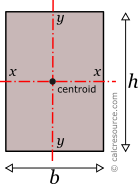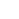Rectangular Section Calculator

This tool calculates the properties of a rectangular cross-section. Enter the shape dimensions 'b' and 'h' below. The calculated results will have the same units as your input. Please use consistent units for any input.

 b = h = Geometric properties: Area = Perimeter = Properties related to x-x axis: Ix = Sx = Zx = Rgx = ADVERTISEMENT Properties related to y-y axis: Iy = Sy = Zy = Rgy = Other properties: Iz =Definitions

Geometry

The area A and the perimeter P of a rectangular cross-section, with sides b and h, can be found with the next formulas:

Moment of Inertia

The moment of inertia (second moment of area) of a rectangle around an axis x, passing through centroid, and being parallel to its base b, is given by the following expression:

where b is the base width, and specifically the dimension parallel to the axis, and h is the height (more specifically, the dimension perpendicular to the axis).

The moment of inertia (second moment of area) of a rectangle around a centroidal axis y, perpendicular to its base, b (in other words parallel to its height, h), can be found, by an equation similar to the last one, but with dimensions b and h interchanged:

Moment of inertia and bending

The moment of inertia (second moment or area) is used in beam theory to describe the rigidity of a beam against flexure. The bending moment M, applied to a cross-section, is related with its moment of inertia with the following equation:

where E is the Young's modulus, a property of the material, and the curvature of the beam due to the applied load. Therefore, it can be seen from the former equation, that when a certain bending moment M is applied to a beam cross-section, the resulting curvature is reversely proportional to the moment of inertia I.

The dimensions of moment of inertia are .

Polar moment of inertia

The polar moment of inertia, describes the rigidity of a cross-section against torsional moments, likewise the planar moments of inertia, described above, are related to flexural bending. The calculation of the polar moment of inertia around an axis z (that is perpendicular to the section plane), can be done with the Perpendicular Axes Theorem:

where the and are the moments of inertia around axes x and y, which are mutually perpendicular with z and meet at a common origin.

Elastic modulus

The elastic modulus of any cross section around axis x (centroidal), describes the response of the section under elastic flexural bending. It is defined as:

where the moment of inertia of the section around x axis and the distance from centroid, of a section fiber, parallel to the x axis and measured perpendicularly from it. Typically, the more distant fiber(s) are of interest. For the a rectangle, the most distant fibers, from the x axis, are those at the top and bottom edge b, with a distance equal to h/2. Therefore, application of the above formula for the rectangular cross-section results in the following expression for elastic section modulus around x axis:

Similarly, for the section modulus , defined around y axis, the most distant fibers from the axis, are the side edges h, with a distance equal to b/2. Application of the general formula leads to the following elastic modulus around the y axis:

One can observe, that the formula for , becomes identical to that for , if we swap b for h and vice versa.

Elastic stresses

If a bending moment is applied on x axis, the section will respond with normal stresses, varying linearly with the distance from the neutral axis (which under elastic regime coincides with centroidal x-x axis). Over neutral axis the stresses are zero. Absolute maximum will occur at the most distant fiber, with magnitude given by the formula:

From the last equation, the section modulus can be considered for flexural bending, a property analogous to cross-sectional A, for axial loading. For the latter, the normal stress is F/A.

The dimensions of section modulus are .

Plastic modulus

The plastic section modulus is similar to the elastic one, but defined with the assumption of full plastic yielding of the cross section due to flexural bending. In that case the whole section is divided in two parts, one in tension and one in compression, each under uniform stress field. For materials with equal tensile and compressive yield stresses, this leads to the division of the section into two equal areas, , in tension, and , in compression, separated by the neutral axis. This is a result of equilibrium of internal forces in the cross-section, under plastic bending. Indeed, the total compressive force, over the entire compressive area, would be , assuming plastic conditions (i.e. the material would have yielded everywhere) and that the compressive yield stress is equal to . Similarly, the tensile force would be , using the same assumptions. Enforcing equilibrium:

The axis is called plastic neutral axis, and for non-symmetric sections, is not the same with the elastic neutral axis (which again is the centroidal one). Rectangular section however, is a symmetrical one (indeed it features two axes of symmetry) and therefore its plastic neutral axes coincides with the elastic ones. In other words, the plastic neutral axes pass through the centroid of the rectangle.The plastic neutral axis divides the cross section in two equal areas, provided the material features equal tensile and compressive yield strength.

Around x axis

The plastic modulus, for bending around the x axis, is given by the general formula:

where , the distance of the centroid of the compressive area from the plastic neutral axis and the respective distance of the centroid of the tensile area.

For the case of a rectangular cross-section, the plastic neutral axis passes through centroid, dividing the whole area into two equal parts. The compressive area would be a rectangle with dimensions b and h/2. Its centroid would be at a distance equal to h/4, from the x axis, as shown in the next figure. The same applies for the tensile area, too. Therefore, the plastic section modulus, of the rectangular section, under x-x bending, is found like this:

which becomes:

Around y axis

With similar considerations, the plastic modulus, of the rectangular section, under y-y bending, can be found through the following formula:

Once again, this last equation can be derived from the plastic modulus , if we swap h for b and vice versa.

Radius of gyration of a cross-section, relative to an axis, is given by the formula:

where I the moment of inertia of the cross-section around the same axis and A its area. The dimensions of radius of gyration are . It describes how far from centroid the area is distributed. Small radius indicates a more compact cross-section. For a rectangular section substitution to the above expression gives the following radii of gyration, around centroidal axes x and y, respectively:

Circle is the shape with minimum radius of gyration, compared to any other section with the same area A.

Rectangular section formulas

The following table, includes the formulas, one can use to calculate the main mechanical properties of the rectangular section.

Related pages

Properties of a Rectangular Tube
Properties of a Circular section
Properties of a Rounded Rectangle section
Moment of Inertia of a Rectangle
All Cross Section tools